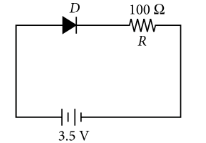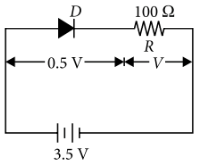P-N junction diode
Question

# In the given figure, a diode D is connected to an external resistance R = 100 $\Omega$ and an e.m.f. of 3.5 V. If the barrier potential developed across the diode is 0.5 V, the current in the circuit will beModerate
Solution

##The potential difference across the resistance  R  is By Ohm's lawThe current in the circuit is

Get Instant Solutions